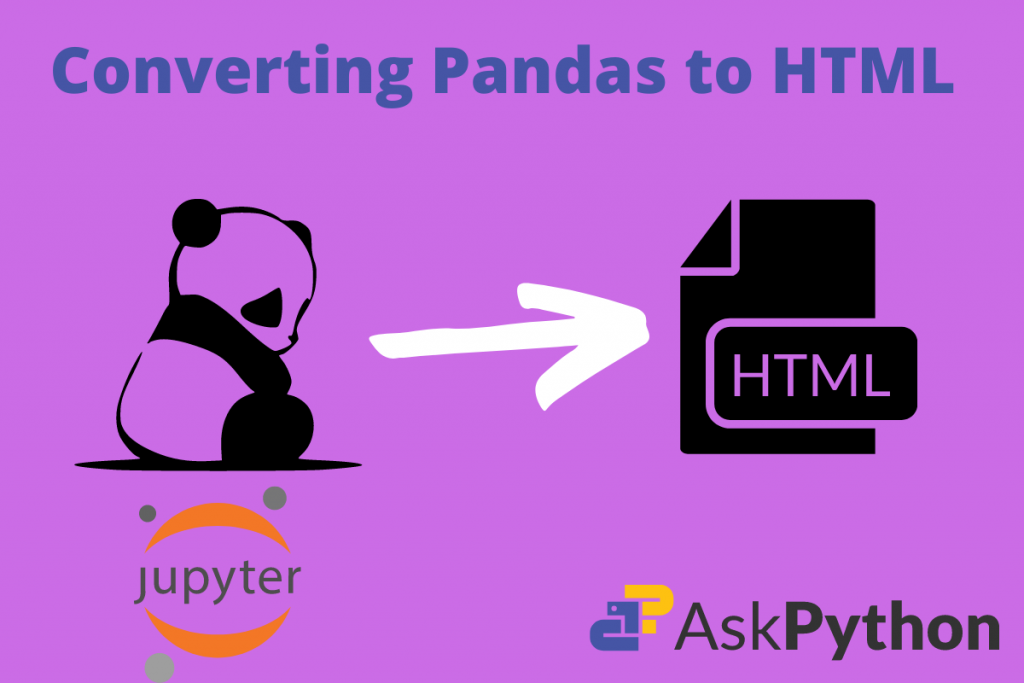# Displaying a data frame in HTML format in PandasIn this article, we will be discussing some of the core functionalities in the Pandas module. If some data science enthusiasts are reading this article then they have got an easy-to-read article this time. But, those who are new to Python and Pandas need some core knowledge of this Python package.

## Getting started with displaying Pandas dataframes in HTML

Our task is to learn how can we convert a basic data frame in the HTML format. These will be the following steps we will be covering in this article:

1. Creating/importing a basic data frame in Pandas.
2. Printing its information.
3. Converting it to HTML.
4. What to do with that format?
5. How to view it in a browser?

### 1. Creating a basic data frame in Pandas

A data frame in Pandas is a tabular representation of data elements that are interconnected to each other. A change in one reflects in another. So, to create it we need to pass a Python Dictionary to the pd.DataFrame() function and our work are easy for further process.

```import pandas as pd
data_dict = {'Name':['Ramesh', 'Suresh', 'Rajeev', 'Neha', 'Aditi'],
'Roll_nos':[1, 2, 3, 4, 5],
'Division':['A', 'B', 'C', 'D', 'E'],
'Percentages':[82, 76, 91, 95, 89]
}

data = pd.DataFrame(data_dict)
data
```Creates a basic dataframe that holds student data

Code explanation:

1. Import the pandas module
2. Create a simple Python dictionary that has 4 columns:
1. Name
2. Roll number
3. Division
4. Percentages
3. Pass that dictionary into the DataFrame() function. Assign a variable to it as data.
4. Then call that variable which will print the it in the tabular format.

### 2. Printing basic information

Then try to print the basic information of this data frame. This is simple with the dataframe.describe method.

```data.columns # displays a list of columns in the data frame
data.info()  #  displays the basic info related to data frame
data.describe() # describes the basic statistical data
```Displaying the basic info of the data frame

### 2. Converting it to HTML format

Pandas module has an HTML function to deliver the given data frame in the respective code. The name of this function is to_html(). The main priority of this function is we need to call it using the dot (‘ . ‘) operator with our respective data frame name. Example: DataFrame.to_html(). Let us apply this to ours’s also.

```data_html = data.to_html()
print(data_html)
```

Code explanation:

1. Create a data_html variable. In that call the to_html function with respect to our data frame.
2. Call that variable.

This generates the following code in HTML format:

```<table border="1" class="dataframe">
<tr style="text-align: right;">
<th></th>
<th>Name</th>
<th>Roll_nos</th>
<th>Division</th>
<th>Percentages</th>
</tr>
<tbody>
<tr>
<th>0</th>
<td>Ramesh</td>
<td>1</td>
<td>A</td>
<td>82</td>
</tr>
<tr>
<th>1</th>
<td>Suresh</td>
<td>2</td>
<td>B</td>
<td>76</td>
</tr>
<tr>
<th>2</th>
<td>Rajeev</td>
<td>3</td>
<td>C</td>
<td>91</td>
</tr>
<tr>
<th>3</th>
<td>Neha</td>
<td>4</td>
<td>D</td>
<td>95</td>
</tr>
<tr>
<th>4</th>
<td>5</td>
<td>E</td>
<td>89</td>
</tr>
</tbody>
</table>
```

Thus we have successfully converted our Pandas data frame to HTML format.

### 4. What to do with that format?

Now a question might arise what is the use of this format.

We can insert this in our web page or any website where we want to display some table of contents.

In fact, anyone who wants to display some of the data in tabular form does not need to create such a big HTML code. He can just write a few lines of code in Python and his work is over.

### 5. How to view it in a browser?

This is HTML code, so we need to know what its outputs are. So, we can make things easier.

1. Copy and paste this code in a raw document and save the extension as .html.
2. Then open it in your favorite browser.
3. This is the output: